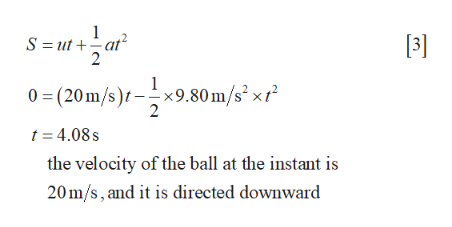# A ball is thrown from the top of a building with an initial velocity of 20.0 m/s straight upward, at an initial height of 50.0 m above the ground.  The ball just misses the edge of the roof on its way down.  Determine (a) the time needed for the ball to reach its maximum height, (b) the maximum height, (c) the time needed for the ball to return to the height from which it was thrown and the velocity of the ball at the instant, (d) the time needed for the ball to reach the ground, and (e) the velocity and position of the ball at t=5.00 s.  Neglect air drag.

Question
879 views

A ball is thrown from the top of a building with an initial velocity of 20.0 m/s straight upward, at an initial height of 50.0 m above the ground.  The ball just misses the edge of the roof on its way down.  Determine (a) the time needed for the ball to reach its maximum height, (b) the maximum height, (c) the time needed for the ball to return to the height from which it was thrown and the velocity of the ball at the instant, (d) the time needed for the ball to reach the ground, and (e) the velocity and position of the ball at t=5.00 s.  Neglect air drag.

check_circle

star
star
star
star
star
2 Ratings
Step 1

(a). Write the kinematic equation and solve for time needed for the ball to reach its maximum height.

Step 2

(b). Write the kinematic equation and solve for maximum height.

Step 3

(c). Write the kinematic equation and solve for time needed for t...help_outlineImage Transcriptionclose3] S ut at 2 0 (20m/s)x9.80 m/s2xr 2 t 4.08s the velocity of the ball at the instant is 20m/s, and it is directed downward fullscreen

### Want to see the full answer?

See Solution

#### Want to see this answer and more?

Solutions are written by subject experts who are available 24/7. Questions are typically answered within 1 hour.*

See Solution
*Response times may vary by subject and question.
Tagged in

### Physics